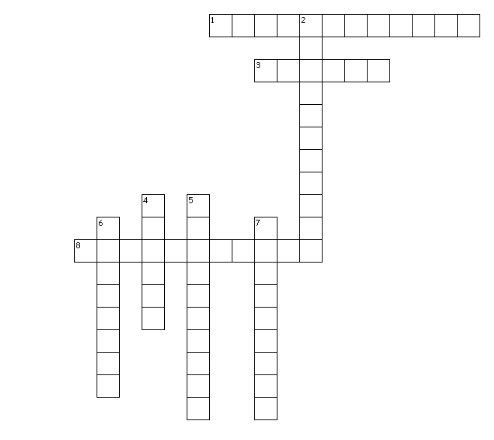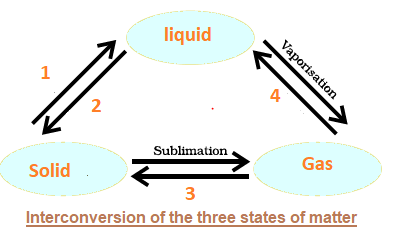# Important questions for matter in our Surroundings

Given below are the Class 9 Science CBSE Important questions for matter in our Surroundings
ope you like them and do not forget to like , social share and comment at the end of the page.

Question 1. What is condensation? How is the condensation of a gas carried out?

Question 2. Why do solids not diffuse?

Question 3. Convert the following Kelvin temperature to degrees Celsius.
a. 175 K
b. 295 K
c. 300 K
d. 225 K

Question 4. Convert the following Celsius temperature to Kelvin temperature.
a. 25 0C
b. -15 0C
c. 0 0C
d. 3 0C

Question 5. Arrange the following substances in increasing order of intermolecular force of attraction:
water, sugar, oxygen

Question 6.  What is the physical state of water at the following temperatures?
(a) 25 0C
(b) 0 0C
(c) 100 0C

Question 7. Why does the temperature of a substance remain constant during melting and boiling even when heat is being supplied to it continuously?

Question 8. Explain the diffusion of copper sulphate into water.

Question 8. Why do the gases exert more pressure on the walls of the container than the solids?

Question 9. The process in which a solid is converted directly into a gas is called sublimation. Iodine is an element that sublimes. A sample of solid iodine in a stoppered flask was allowed to stand undisturbed for several days. Crystals of solid iodine grew on the sides of the flask. Explain at the molecular level what happened?
Question 10. Give three examples of crystalline and amorphous solids.

Question 11. Why is motor oil more viscous than water? Does motor oil have a greater surface tension than water.
Question 12. Describe why a drop of food coloring in a glass of water slowly becomes evenly distributed without the need for stirring?
Question 13. Liquid mix more slowly than gases. Why?
Question 14. Define the following terms:
a. Melting point
b. Freezing point
c. Boiling point

## Foundation Level Questions for Olympiads/JEE Level/PMT/KVYP

Question 15. A constant pressure tank of gas at 1.01 Atm has propane in it at 150C when it is at 255 cubic meters. What is its volume at 480C?

Question 16. What is the mass of 15 liters of chlorine gas at STP?

Question 17. How many liters of hydrogen at 00C and 1400mm of Hg are produced if 15g of magnesium reacts with sulphuric acid?

Question 18. A 250 Kg tank of liquid butane (C4H10) burns to produce carbon dioxide at 1200C. What volume of carbon dioxide is produced at 1 Atm?

Question 19. How many liters of product at 950 mmHg and 00C is produced by the burning of three liters of acetylene (C2H2) at 5 atm and 200C?

Question 20. A fixed quantity of gas at 230C exhibits pressure of 748 torr and occupies a volume of 10.3 L
a)Use Boyle's law to calculate the volume the gas will occupy at 230C if the pressure is increased to 1.88 atm.
b)Use Charles' law to calculate the volume the gas will occupy if the temperature is increased to 1650C while the pressure is held constant.

Question 21. (a) Write the ideal- gas equat ion, and give the units used for each term in the equation when
Given that R = 0.0821 L-atm/mol- K
(b) What is an ideal gas?

Question 16. A certain volume of a gas is under a pressure of 900 mm of Hg. When the pressure is increased by 300 mm, the gas occupies 2700 ml. if this change occurs at a constant temperature, calculate the initial volume of the gas.
Question 17. Which state of matter is compressible? Why?

Question 22. At a pressure of 2 atmosphere a fixed mass of hydrogen occupies a volume of 8 liters. What pressure must be maintained if the volume is to be increased to 10 liters, temperature remaining constant?
Question 23. A given mass of a gas occupies 960 ml at 270C C. What volume will it occupy if the temperature is raised to 1770C C, pressure remaining constant?
Question 24.
Crossword PuzzleAcross
1. BEC stands for Bose-Einstein-______
3. The state consists of super energetic and super excited particles
8. Conversion of solid to vapour is called ______
Down
2. This is the phenomenon of change of a liquid into vapours at any temperature below its boiling point
4. SI unit of Temperature
5. CNG stands ____ natural gas
6. It is the amount of water vapour present in air.
7. LPG stands for ______petroleum gas.

Question 25.
Write the terms for numbers in the below figureLatest Articles
Synthetic Fibres and Plastics Class 8 Practice questions

Class 8 science chapter 5 extra questions and Answers

Mass Calculator

3 Fraction calculator

Garbage in Garbage out Extra Questions7